# Multiplication Tables

## Maths times table games

Here, we provide math times table games for students, math times table questions to do practice, maths time tables test to check your tables skills, math times tables practice quiz for all.

Select Table :

1
2
3
4
5
6
7
8
9
10
11
12
13
14
15
16
17
18
19
20
2  x  1  =
2  x  2  =
2  x  3  =
2  x  4  =
2  x  5  =
2  x  6  =
2  x  7  =
2  x  8  =
2  x  9  =
2 x 10  =

### Why do we learn maths ?

Mathematics is very important in our daily life. It’s a gate and key to the sciences. Without mathematics, there is nothing we can do. Everything around us it mathematics and numbers. Math equips us with problem-solving skills that help you analyze real-life situations and arrive at logical solutions based on the given data. It teaches us many significant skills that we use every single day without even realizing it. We can ensure that it is next to impossible to live an independent life without basic math skills.

### Math table practice (Math times table questions)

Multiplication is a fundamental skill that will enable us to calculate the number faster than addition. Without it chid can’t learn other concepts of maths like algebra and fractions. It’s simply a Rapid way of adding the same number over and over which is why it is also known as repeated addition.

It’s an important topic in learning maths after addition and subtraction. Children learn multiplication tables in elementary school. Without this, Math becomes quite difficult to understand. This is the reason why children are made to learn these in elementary school. How well the child learns the multiplication tables has a direct effect on how they learn and make progress in maths. Besides being useful for elementary kids, these have greater use in the later years of a person’s life.

When children learn to recall the time’s tables immediately without having to think much, this will have a great impact on their confidence. They will get praise from his teachers and fellow students. This will go a long way in building up their confidence and interest in mathematics. Multiplication tables help them to solve problems faster. It gives them a sense of satisfaction and this makes them happier.

Shiv Kids Education is offered here Online math times table questions for class 1 to class 6 students. As we know, addition, subtraction, multiplication, and division are the basic concepts of Mathematics. Students commence their basic maths learning through these concepts in school. Nowadays rarely we get a free source of Online Math Practice for students over the internet. There are several good paid websites available for it but here any users can do free practice as much as they want.

Click on the following image link to check out other pages of math times table quiz.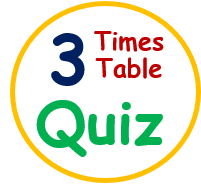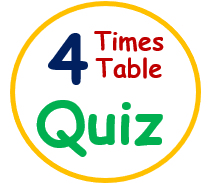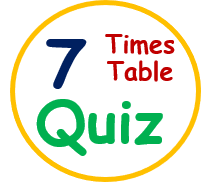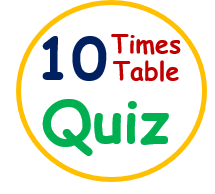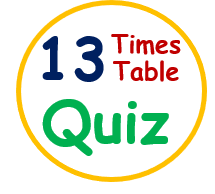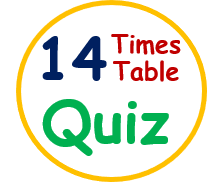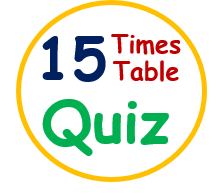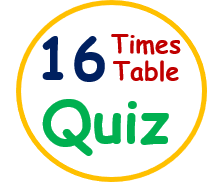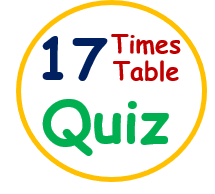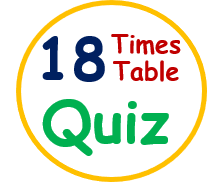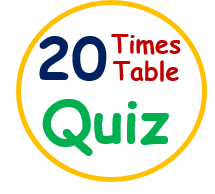Enjoy here other relevant topics as well by clicking on the following links.